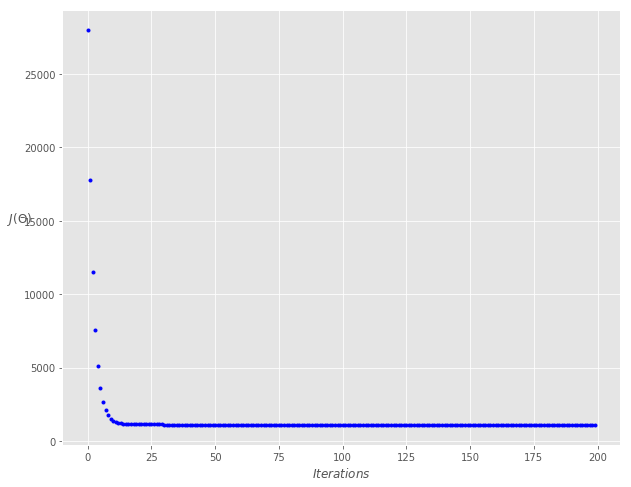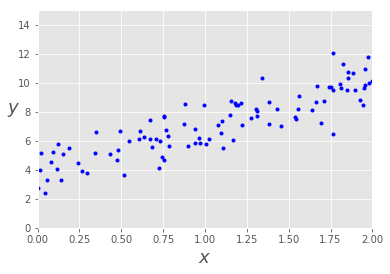$$y = b + mx$$

$$J(\theta) = \theta_0 + \theta_1 x$$

array([[3.86687149],
[3.12408839]])


array([[ 3.86687149],
[10.11504826]])


Cost

$$\begin{equation} J(\theta) = 1/2m \sum_{i=1}^{m} (h(\theta)^{(i)} - y^{(i)})^2 \end{equation}$$

$$\begin{equation} \frac{\partial J(\theta)}{\partial \theta_j} = 1/m\sum_{i=1}^{m}(h(\theta^{(i)} - y^{(i)}).X_j^{(i)} \end{equation}$$

\begin{equation}
\theta_0: = \theta_0 -\alpha . (1/m .\sum_{i=1}^{m}(h(\theta^{(i)} - y^{(i)}).X_0^{(i)})
\end{equation}

\begin{equation}
\theta_1: = \theta_1 -\alpha . (1/m .\sum_{i=1}^{m}(h(\theta^{(i)} - y^{(i)}).X_1^{(i)})
\end{equation}

\begin{equation}
\theta_2: = \theta_2 -\alpha . (1/m .\sum_{i=1}^{m}(h(\theta^{(i)} - y^{(i)}).X_2^{(i)})
\end{equation}

\begin{equation}
\theta_j: = \theta_j -\alpha . (1/m .\sum_{i=1}^{m}(h(\theta^{(i)} - y^{(i)}).X_0^{(i)})
\end{equation}

Theta0:          3.867,
Theta1:          3.124
Final cost/MSE:  5457.747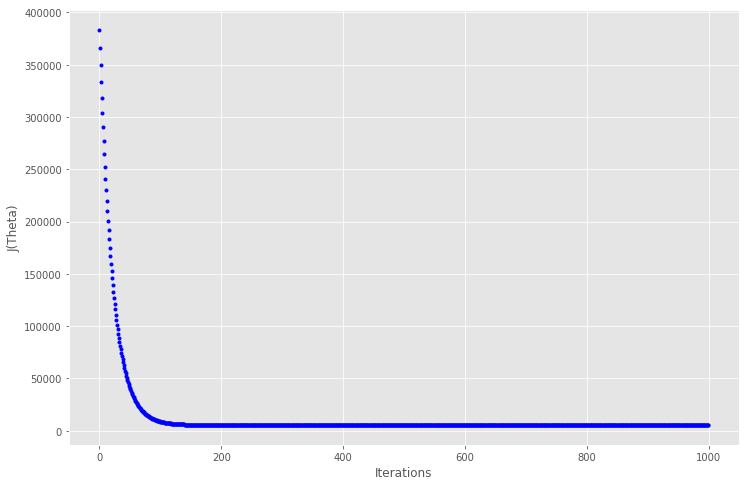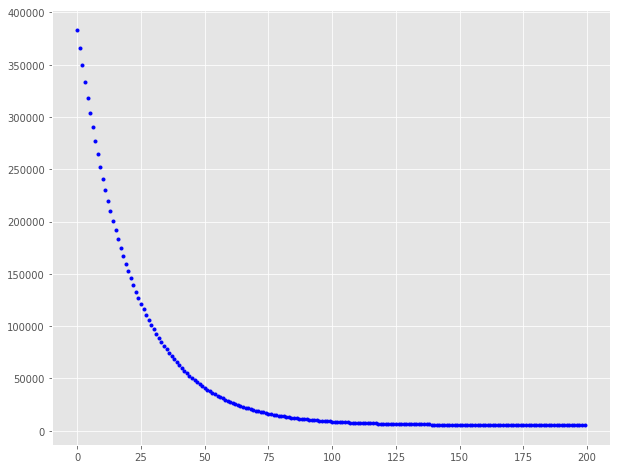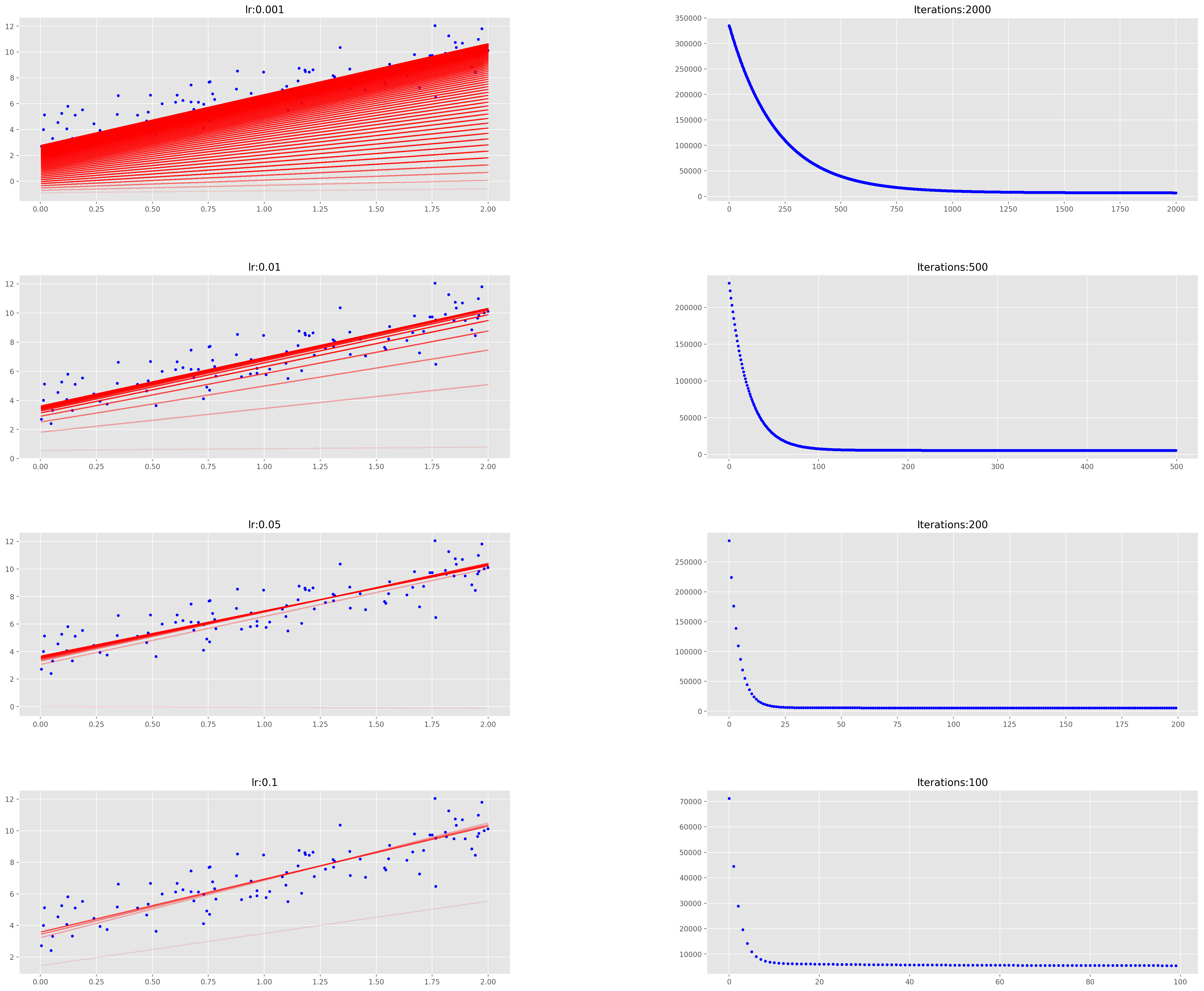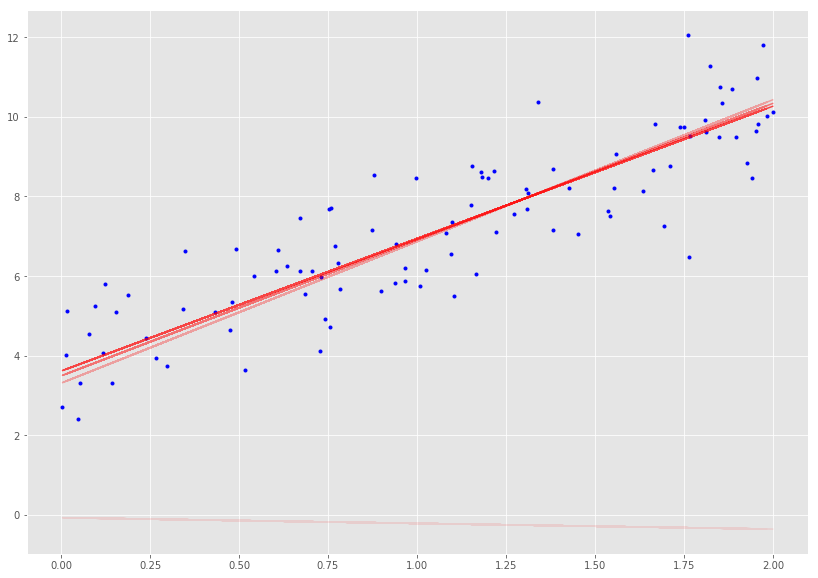$$\theta_i = \theta_i - \alpha (h_\theta(x_0^{(j)}, x_1^{(j)}, …x_n^{(j)}) - y_j)x_i^{(j)}$$

Theta0:          3.762,
Theta1:          3.159
Final cost/MSE:  46.964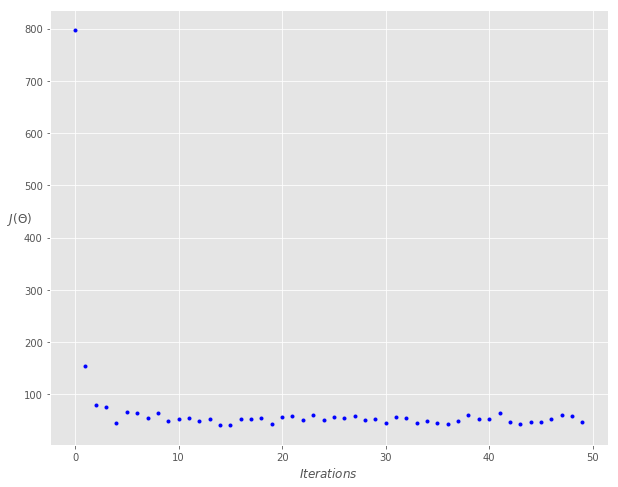$$\theta_i = \theta_i - \alpha \sum\limits_{j=t}^{t+x-1}(h_\theta(x_0^{(j)}, x_1^{(j)}, …x_n^{(j)}) - y_j)x_i^{(j)}$$

Theta0:          3.842,
Theta1:          3.146
Final cost/MSE:  1090.518